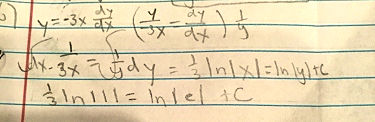Search IntMath
Close

# Differential equation: separable [Solved!]

### My question

Y=-3xdy/dx and y(1)= e

### Relevant page

2. Separation of Variables

### What I've done so farX

Y=-3xdy/dx and y(1)= e
Relevant page

<a href="/differential-equations/2-separation-variables.php">2. Separation of Variables</a>

What I've done so far## Re: Differential equation: separable

Hello, Struggling

First, be careful with upper- and lower-case variables - they mean different things.

I believe your question is actually y=-3xdy/dx and not Y=-3xdy/dx.

Also, you are encouraged to use the math entry system here - it's easier than uploading a photo!

In your second line, the negative is hard to read. I gather this is what you mean:

int 1/(-3x) dx = int 1/y dy

Also be careful with the "=" sign. The one in the middle of your second line is "dangerous", since the expression immediately to the left of it will not necessarily be equal to the one immediately to the right when solving DEs. (Here, it could be correct, if the negative wasn't dropped.) It's best to write a new line.

The last part of the second line has a problem. Where did the negative go?

X

Hello, Struggling

First, be careful with upper- and lower-case variables - they mean different things.

I believe your question is actually y=-3xdy/dx and not Y=-3xdy/dx.

Also, you are encouraged to use the math entry system here - it's easier than uploading a photo!

In your second line, the negative is hard to read. I gather this is what you mean:

int 1/(-3x) dx = int 1/y dy

Also be careful with the "=" sign. The one in the middle of your second line is "dangerous", since the expression immediately to the left of it will not necessarily be equal to the one immediately to the right when solving DEs. (Here, it could be correct, if the negative wasn't dropped.) It's best to write a new line.

The last part of the second line has a problem. Where did the negative go?

## Re: Differential equation: separable

Integrating both sides of

int 1/(-3x) dx = int 1/y dy

gives

-1/3 ln|x| = ln|y| + K (including the - sign that was missing from the OP's answer)

The question says y(1)= e, so to find the value of K we substitute:

-1/3 ln|1| = ln|e| + K

ln|1| = 0 and ln|e| = 1, so we have

0 = 1 + K, so

K=-1

-1/3 ln|x| = ln|y| - 1

We can express it in terms of y fairly easily:

Add 1 to both sides:

-1/3 ln|x| + 1 = ln|y|

Since log(a^b) = b log a, we can write:

ln|x^(-1/3)| + 1 = ln|y|

and ln(e) = 1, so we can put:

ln|x^(-1/3)| + ln(e) = ln|y|

Using log(ab) = log(a) + log(b), we can combine the LHS:

ln|ex^(-1/3)| = ln|y|

Taking "e to both sides" (i.e., finding the antilogarithms), we have:

y = ex^(-1/3)

X

Integrating both sides of

int 1/(-3x) dx = int 1/y dy

gives

-1/3 ln|x| = ln|y| + K (including the - sign that was missing from the OP's answer)

The question says y(1)= e, so to find the value of K we substitute:

-1/3 ln|1| = ln|e| + K

ln|1| = 0 and ln|e| = 1, so we have

0 = 1 + K, so

K=-1

-1/3 ln|x| = ln|y| - 1

We can express it in terms of y fairly easily:

Add 1 to both sides:

-1/3 ln|x| + 1 = ln|y|

Since log(a^b) = b log a, we can write:

ln|x^(-1/3)| + 1 = ln|y|

and ln(e) = 1, so we can put:

ln|x^(-1/3)| + ln(e) = ln|y|

Using log(ab) = log(a) + log(b), we can combine the LHS:

ln|ex^(-1/3)| = ln|y|

Taking "e to both sides" (i.e., finding the antilogarithms), we have:

y = ex^(-1/3)

## Re: Differential equation: separable

As usual, a good quality answer, stephenB.

X

As usual, a good quality answer, stephenB.

You need to be logged in to reply.

## Related Differential Equations questions

• Re: ODE [Pending...]
You haven't shown any working so I can't see where you are getting stuck. Your...
• polygons [Solved!]
Trying to find the area of a honey comb. My answer does not equal...
• Differential equation - has y^2 [Solved!]
I am seraching a solution of: c1*y^2+c2+dy/dx = 0 c1 and c2 are constants
• dy/dx = xe^(y-2x), form differntial eqaution [Solved!]
dy/dx = xe^(y-2x) , i am asked to form differential equation using this equation ....
• ODE seperable method [Solved!]
(y/x)*(dy/dx)=sqrt(1+x^2+y^2+x^2*y^2)